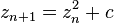# Patterns

This piece is called Looking Through.“Looking Through”
Digital Art printed on metal, single edition
20×20″
\$345.00 Artist: Lianne Todd

Looking through what?  A microscope?  A telescope? A porthole?

In a fractal universe, it doesn’t really matter.  Similar patterns are present on multiple scales.  Use your imagination!

This image is actually a combination of fractals – one for the thing we are looking through (the self-similarity on smaller scales provides the illusion of perspective and depth here), and one for what we are looking at (this is a flame fractal – more about them later).

As illustrated here, fractal geometry is quite versatile.  I’ve seen some discussion on ‘true’ fractals versus ‘near’ fractals and I would like to address that here for a moment.  There seems to be an opinion out there that for a fractal to be ‘true’ it must be a)infinite and b)exactly the same no matter what scale you look at.  Having read most of Benoit Mandelbrot’s Fractal Geometry of Nature, I have a problem with these stipulations.  First of all, the equation for Mandelbrot’s set is$z_{n+1}=z_n^2+c$, with as the number of iterations, where c is a complex parameter.

There is more to explaining the Mandelbrot set than that, of course, but that is the equation, and if n is a given number, then it’s not infinite, is it?  Perhaps the possibility of an infinite number of iterations exists, but that’s an argument for another day.

And even Mandelbrot’s set is not exactly the same on multiple scales. The PATTERN is there, it’s just slightly altered at different scales.  It is self-similar.  This is one of the things which makes fractal geometry so suitable for modelling the universe.

In my understanding, there was never a suggestion by Mandelbrot, the founder of fractal geometry, that a “true” fractal had to be infinite OR exactly the same on multiple scales.  Rather, a fractal is strictly defined as “a set for which the Hausdorff Besicovitch dimension strictly exceeds the topological dimension.”

So, perhaps I’m getting it all wrong, but if you would like to argue I would welcome your discussion.

And now, because I was once a biologist and if you’re anything like me you need a more highly magnified look at that thing, here is a zoom of what you were “looking through” at:## 2 thoughts on “Patterns”

1. Lianne. You are exactly right that Mandelbrot never said that a “true” fractal needs to be infinite and exactly the same at every scale. There are some fractals that are exactly the same at every scale such as the Koch Curve or Sierpinski Gasket. You can imagine this going on to infinity in both directions. However, because these fractals are generated on a computer, with a limit to its digits of precision, even these fractals are not infinite. The Mandelbrot set is a different kind of fractal than the self-same fractals. It similar (self-similar) but DIFFERENT at all scales. It is also subject to the limit to the digits of precision of the computer so at some very small scale, the fractal breaks down. Does this mean that the Mandelbrot Set is not a “true” fractal? Absolutely not. The Mandelbrot set is the most “true” fractal of all the fractals I have studied. If the computer had more digits of precision (more bits) then you would find more fractals down there. That said, the Universe also has a limit to its digits of precision called the Planck Limit. This limits the scale with which fractals can be generated in the Universe. It common knowledge (according to the holographic principle) that fractal patterns break down at the Planck limit. If the Universe were a fractal (and I believe it is), it is more like the Mandelbrot fractal (ie. self-similar at different scales) and less like the self-same fractals of the Koch and Sierpinski curves.

Like

This site uses Akismet to reduce spam. Learn how your comment data is processed.